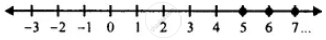# For the Following Inequation, Represent the Solution on a Number Line : 3(2x -1) ≥ 2(2x + 3), X ∈ Z - Mathematics

Sum

For the following inequation, represent the solution on a number line :

3(2x -1) ≥ 2(2x + 3), x ∈ Z

#### Solution

3(2x -1) ≥ 2(2x + 3), x ∈ Z

⇒ 6x - 3 ≥ 4x + 6, x ∈ Z

⇒ 6x - 4x ≥ 6 + 3

⇒ 2x ≥ 9

⇒ x ≥ 9/2

⇒ x ≥ 4 1/2

∴ x = {5, 6, 7, ....}Is there an error in this question or solution?

#### APPEARS IN

Selina Concise Mathematics Class 8 ICSE
Chapter 15 Linear Inequations
Exercise 15 (B) | Q 13.2 | Page 175